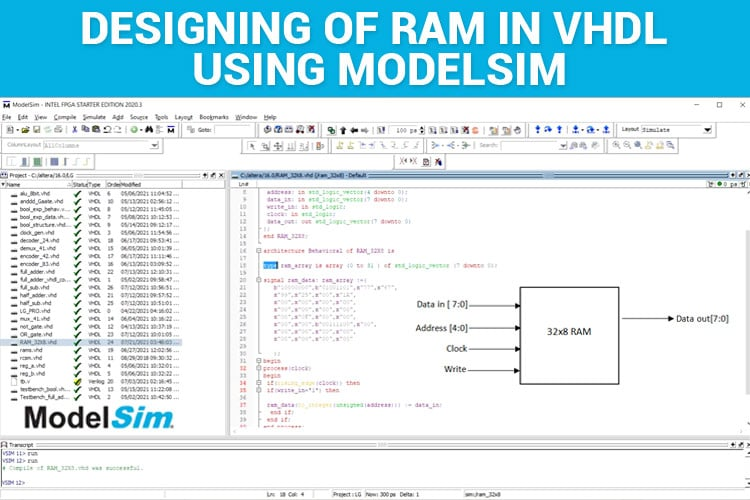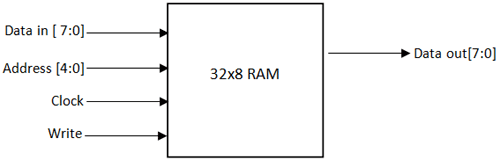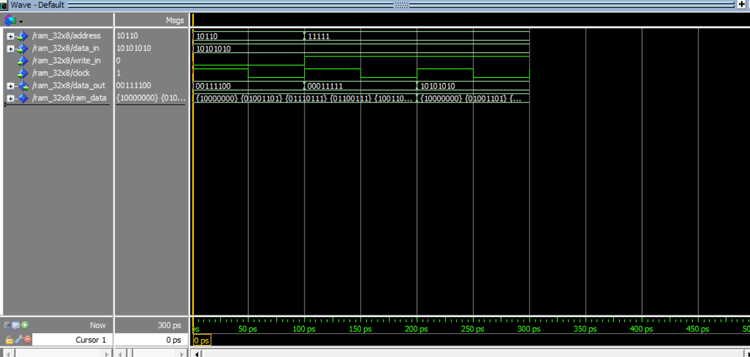# Designing of RAM in VHDL using ModelSim

Published  August 19, 2021   0
R
AuthorIf you had gone down to a nearby laptop store for purchasing one, you would have come across the terms “DDR4 DDR3”. For those who are unfamiliar with "DDR4 DDR3", these are different versions of RAM (Random Access Memory). We users always look for better versions of RAM such that there is a seamless performance by the system. Guess what, in this tutorial we are going to explore how to implement the RAM in VHDL using ModelSim. If you are new here, you can checkout our previous VHDL tutorials using ModelSim.

### What is a RAM (Random Access Memory)?

RAM (Random Access Memory) is a memory device that is used in the systems read/write and store the data. RAM is present ranging from small-scale systems such as embedded systems, smartphones to large-scale systems as Desktop, Laptops, etc. The RAM used in a system has a major impact on the system's performance. It's responsible for carrying out multiple tasks and storing data. The higher the version of a RAM, the higher it’s performance.

Now that we have the clear idea about what RAM is, let’s see how to implement RAM in VHDL. Though there are higher versions of RAM that are used in the industry. In this tutorial, we are going to implement a simple 32X8 RAM. It’s a small-scale version of the memory device.

### Specifications of RAM

Usually, memory is represented by M x N, where M is the number of locations and N is the Data lines. So here 32 x 8, “32” represents 32 locations, or an array that has 32 locations, “8” represents 8 data lines. In simpler terms, a 32 x 8 RAM has 32 locations and every 32 locations can store 8-bit data. So, the total number of bits that can be stored by a 32 x 8 RAM is 256 bits. Now, you can imagine the storage capacity of 8GB, 16GB RAM that’s used in laptops/desktops.

Given below is a schematic diagram that indicates the ports/lines in a RAM.A RAM has a data line, address line, write signal, clock signal, and data out port to read the contents from the RAM. The address line size/bits varies depending on M x N specifications. Let's undersatnd how to calculate the size of the address line bits (A).

Address Line bits/size (A): 2A=M. So, in our case, it is 2A=32 (M=32), where A=5.
M → Number of Locations
N → Data Lines
W → Write signal

Since RAM is a sequential circuit, it has a clock signal. All sequential circuits have a clock signal. Also, the write signal takes care of burning the data into the RAM. Only when both clock and the write signal is “1” the data is stored in the RAM.

The events that will take place concerning the write and clock signals are given below.

 Clock Write Signal Event 0 0 No data loaded 0 1 No data loaded 1 0 No data loaded 1 1 Data will be loaded

Now that we know how the RAM works, let’s begin to implement the same in VHDL.

VHDL code for RAM:

```library IEEE;
use IEEE.STD_LOGIC_1164.ALL;
USE ieee.numeric_std.ALL;
entity RAM_32X8 is
port(
data_in: in std_logic_vector(7 downto 0);
write_in: in std_logic;
clock: in std_logic;
data_out: out std_logic_vector(7 downto 0)
);
end RAM_32X8;
architecture Behavioral of RAM_32X8 is
type ram_array is array (0 to 31 ) of std_logic_vector (7 downto 0);
signal ram_data: ram_array :=(
b"10000000",b"01001101",x"77",x"67",
x"99",x"25",x"00",x"1A",
x"00",x"00",x"00",x"00",
x"00",x"00",x"00",x"00",
x"00",x"0F",x"00",x"00",
x"00",x"00",b"00111100",x"00",
x"00",x"00",x"00",x"00",
x"00",x"00",x"00",x"1F"
);
begin
process(clock)
begin
if(rising_edge(clock)) then
if(write_in='1') then
end if;
end if;
end process;
end Behaviorial;
```

Let's go step by step first so that we can keep track of things. First, let's write the code segment by segment and then combine.

The very first step is to import the library and entity declaration. As like, “use IEEE.std_logic_1164.all”, “use IEEE.numeric_std.ALL” a numeric library is imported which we will be using. The usage of this library will be explained below in the code.

The entity is declared with the label “RAM_32X8”. Next to that, all the input and output ports are declared. Address line (A) of bit size five (5), Data Lines (i.e. N =8), write signal (W), a clock signal, and data out are the corresponding input and output ports as explained earlier.

```library IEEE;
use IEEE.STD_LOGIC_1164.ALL;
USE ieee.numeric_std.ALL;
entity RAM_32X8 is
port(
data_in: in std_logic_vector(7 downto 0);
write_in: in std_logic;
clock: in std_logic;
data_out: out std_logic_vector(7 downto 0)
);
end RAM_32X8;```

Once the entity is declared, we can start with the architecture definition. The architecture is labeled as “RAM_32x8”.

`architecture Behavioral of RAM_32X8 is`

type ram_array is array (0 to 31) of std_logic_vector (7 downto 0): Now, we have to create an array of size 32. So, we declare the variable “ram_array” as a “type ram_array” which is an enumeration type. An enumeration type is nothing, but listing all possible values for a variable. “is array (0 to 31)” represents that the “type ram_array” will be an array of size (M) 32 starting from 0 and ending at 31. These 32 locations should be able to store an 8-bit vector as N=8. So we state that as “of std_logic_vector (7 down to 0)”. So typically ram_arary is an array with 32 locations and each location can store 8 bits.

`type ram_array is array (0 to 31) of std_logic_vector (7 downto 0);`

Now, we have to store this as a signal. So, we declare the “ram_data” as a signal and map it to the array “ram_array”. Now the contents of “ram_array” will be mapped to the signal “ram_data”. So, we have to initialize the contents of the array. So, I have initialized some of the values.

NOTE: We can represent/initialize by binary or hexadecimal. “b” represents binary, “x” represents hexadecimal. However, ModelSim by default converts the representation to binary while simulating.

```signal ram_data: ram_array :=(
b"10000000",b"01001101",x"77",x"67",
x"99",x"25",x"00",x"1A",
x"00",x"00",x"00",x"00",
x"00",x"00",x"00",x"00",
x"00",x"0F",x"00",x"00",
x"00",x"00",b"00111100",x"00",
x"00",x"00",x"00",x"00",
x"00",x"00",x"00",x"1F"```

Since the entire if condition process depends on the clock, we declare by “process(clock)”

```begin
process(clock)
begin```

if (rising_edge(clock)) then: ModelSim has a predefined function “rising_edge()”. “rising_edge” means the point where the transition of signal from low to high. So, only if the low to high transition occurs it will pass on to the next statement.

`if(rising_edge(clock)) then `

if (write_in='1') then: This is simple if condition where only if the signal “write_in” is 1 it will pass on to the next.

`if(write_in='1') then`

ram_data v(to_integer(unsigned(address))) <= data_in: The “ram_data” signal itself is an array, so we have to set the index of the array to which the data has to be stored. The index will accept only a positive integer. The “to_integer()” will convert to the integer value. The “unsigned” is used to convert the binary numbers to unsigned binary numbers. The “IEEE.NUMERIC_STD all” package takes care of the integer conversion.

NOTE: By default, Modelsim will take the given inputs as binary.

If your address is “11111”, ModelSim sometimes interprets “11111” as a negative number as MSB (Most significant bit) is “1”. Hence, it's always advisable to use unsigned(). In the end, when the ModelSim process this statement, it will be ram_data(index). Just that we have converted the given address to a proper index format. Now, the data in the “data_in” signal will be stored to the appropriate “ram_data” index.

As we have used 2 ifs, we have to terminate the if’s by two “end if”s

```end if;
end if;
end process;```

Since the data has to be read out from the RAM by the given address, a “data_out” signal has been declared to read the contents from a specific index.

`data_out <= ram_data(to_integer(unsigned(address)));`

Just copy-paste the above code, compile and simulate the code. For simulation procedures, refer to “Implementation of basic logic gates using VHDL in ModelSim”

I have simulated the above code and a sample waveform is given below. Refer to the video for a detailed explanation of the waveforms.Move the cursor along the waveform to read the contents of the signals. This is how the RAM is scripted in VHDL.

Code

library IEEE;
use IEEE.STD_LOGIC_1164.ALL;
USE ieee.numeric_std.ALL;
entity RAM_32X8 is
port(
data_in: in std_logic_vector(7 downto 0);
write_in: in std_logic;
clock: in std_logic;
data_out: out std_logic_vector(7 downto 0)
);
end RAM_32X8;
architecture Behavioral of RAM_32X8 is
type ram_array is array (0 to 31 ) of std_logic_vector (7 downto 0);
signal ram_data: ram_array :=(
b"10000000",b"01001101",x"77",x"67",
x"99",x"25",x"00",x"1A",
x"00",x"00",x"00",x"00",
x"00",x"00",x"00",x"00",
x"00",x"0F",x"00",x"00",
x"00",x"00",b"00111100",x"00",
x"00",x"00",x"00",x"00",
x"00",x"00",x"00",x"1F"
);
begin
process(clock)
begin
if(rising_edge(clock)) then
if(write_in='1') then